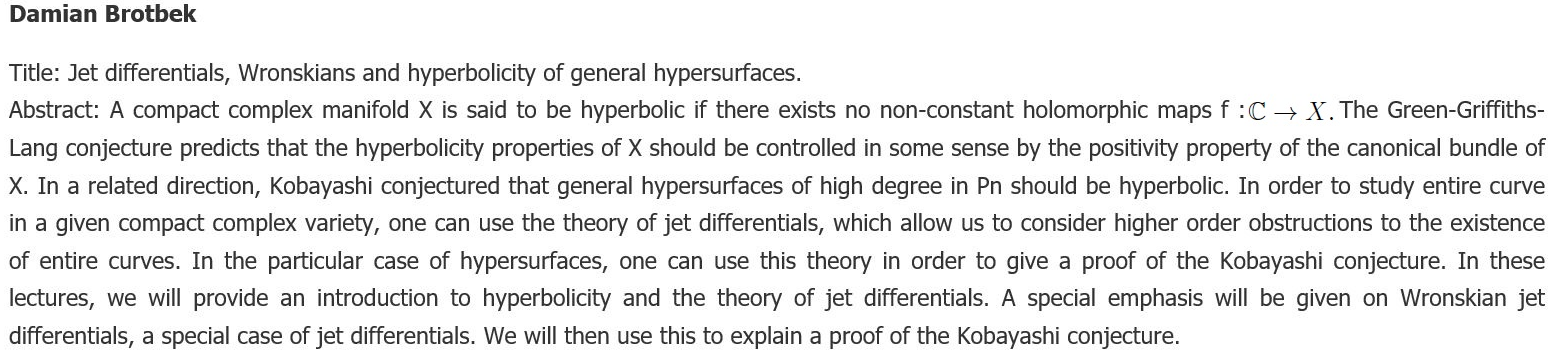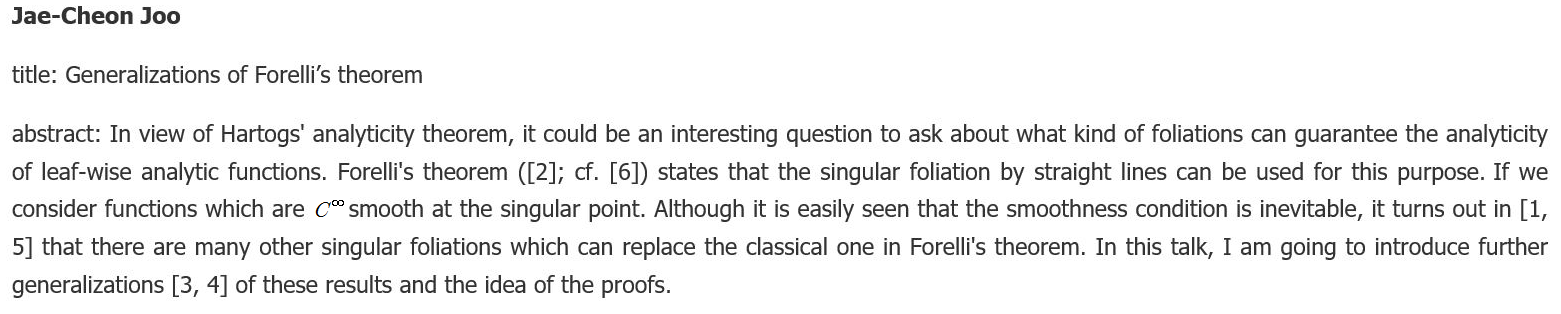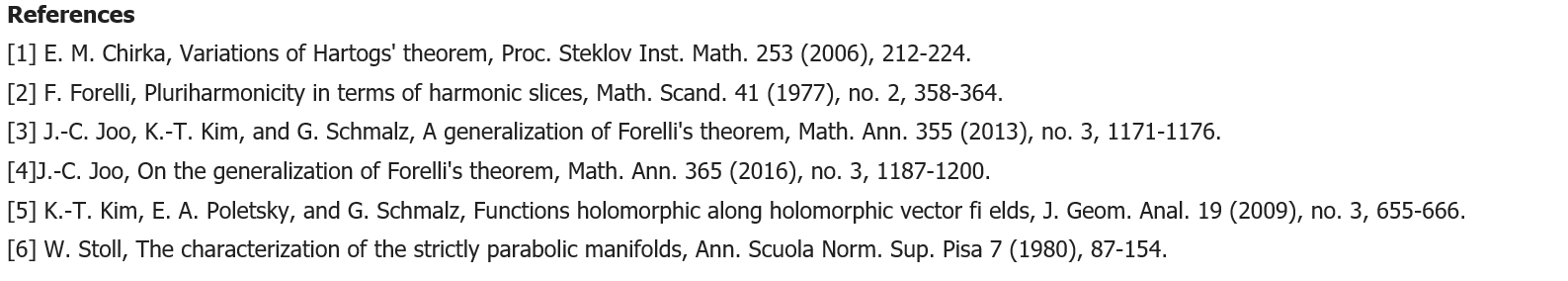﻿ [CMC] progress in several complex variables
 Progress in Several Complex Variables          October 21-25, 2019                                      KIAS 1503
 Title & Abstract Home > Title & Abstract

Bo Berndtsson

Title: Brunn-Minkowski type inequalities and the positivity of vector bundles

Abstract: The first part (roughly one third) of the course will be dedicated to the classical theorem of Brunn-Minkowski and its functional version, Prékopa's theorem. Prékopa's theorem can be proved (following Brascamp and Lieb) from a real variable version of Hörmander's L^2-estimates for the dbar-equation. We will then go on to show how one can obtain complex versions of the theorems from estimates for the dbar-equation. The complex versions concern the positivity of certain Hermitian vector bundles. Finally, we will give some applications to the space of Kähler metrics and the study of fibrations occuring in algebraic geometry.

Lecture Note: (PDF File Download)Young-Jun Choi

Title: Relative Kähler-Ricci flow on a family of strongly pseudoconvex domains

Abstract: In 2012, Schumacher proved that the variation of Kähler-Einstein metrics on a family of canonically polarized compact Kähler manifolds is positive definite on the total space. In his paper, he showed that the geodesic curvature, which measures the positivity of the horizontal direction, satisfies a certain elliptic PDE. Applying the maximum principle to this PDE, he obtained the positivity. In 2013, Berman proved the parabolic version of the Schumahcer's result. More precisely, he proved that the geodesic curvature of a family of canonically polarized compact Kähler manifolds satisfies a parabolic equation. A parabolic maximum principle implies that the positivity of the geodesic curvature is preserved along the Kähler-Ricci flow. In this talk, we will briefly introduce the results of Schumacher and Berman and show how to apply Berman's method to a family of strongly pseudoconvex domains.

Fusheng Deng

Title: Characterizations of plurisubharmonic functions

Abstract: We will discuss characterizations of plurisubharmonic functions in terms of L^p estimates of d-bar or L^p extensions of holomorphic functions. This lecture is based on a series of works joint with Professor Jiafu Ning, Zhiwei Wang, Liyou Zhang, and Xiangyu Zhou.

Lecture Note: (PDF File Download)Kengo Hirachi

Title: Invariant theory for the Szegö kernel

Abstract: Fefferman initiated the invariant theory for the Bergman kernel, which aims to construct all local CR invariants. In this talk, I will formulate a framework for the invariant theory for the Szegö kernel. The goal is to describe local invariants of pseudo-hermitian structure that are invariant under scaling by CR pluri-harmonic functions. As a result, I will give a characterization of pseudo-Einstein contact forms in terms of the vanishing of the log term of the Szegö kernel on 3-dimensional CR manifolds.

Jun-Muk Hwang

Title: Infinitesimal neighborhoods of submanifolds

Abstract: We discuss the rigidity problem of infinitesimal neighborhoods of compact submanifolds of complex manifolds, which goes back to a question of Nirenberg and Spencer asking whether a finite-order infinitesimal neighborhood determines the germ of a submanifold when the normal bundle is positive. Following Hirschowitz's approach, we consider a geometric condition in terms of deformations of the submanifold, replacing the condition on the positivity of the normal bundle. This leads to a reformulation of the problem in terms of families of infinitesimal neighborhoods of submanifolds. We explain some results on this problem with several examples.

References

On the historical side of the problem:

1. Nirenberg, L. and Spencer, D.: On rigidity of holomorphic imbeddings. Contributions to function theory, 133--137, Tata Institute of Fundamental Research, Bombay, 1960

2. Griffiths, P.: The extension problem in complex analysis II; Embeddings with positive normal bundle. Amer. J. Math. 88 (1966) 366--446

3. Morrow, J. and Rossi, H.: Some general results on equivalence of embeddings. Recent developments in several complex variables. Ann. Math. Studies 100, 299--325, Princeton Univ. Press, Princeton, 1981

For the results on projective differential geometry:

4. Griffiths, P.: On Cartan's method of Lie groups and moving frames as applied to uniqueness and existence questions in differential geometry. Duke Math. J. 41 (1974) 775--814

5. Jensen, G.: Higher order contact of submanifolds of homogenoues spaces. Springer Lect. Notes Math. 610, Springer Verlag, Berlin-New York, 1977

6. Verderesi, J. A.: Contact et congruence de sous vari'et'es. Duke Math. J. 49 (1982) 513--515

Related results on K3 surfaces:

7. Beauville, A. and Merindol, J.-Y. : Sections hyperplanes des surfaces K3. Duke Math. J. 55 (1987) 873--878.

8. Ciliberto, C., Lopez, A. and Miranda, R.: Projective degenerations of K3 surfaces, Gaussian maps, and Fano threefolds. Invent. Math. 114 (1993) 641--667

9. Huybrecht, D.: Lectures on K3 surfaces. Cambridge Studies in Advanced Mathematics 158, Cambridge Univ. Press, Cambridge, 2016

On the Cartan equivalence method for G-structures:

10. Singer, I. M. and Sternberg, S.: The infinite groups of Lie and Cartan, Part I, (The transitive groups). J. d'Analyse Math. 15 (1965) 1--114

11. Morimoto, T.: Sur le probl`eme d''equivalence des structures g'eom'etriques. Japan. J. Math. 9 (1983) 293--372

12. Hwang, J.-M.: An application of Cartan's equivalence method to Hirschowitz's conjecture on the formal principle. Ann. Math. 189 (2019) 979--1000Michael Kapovich

Title: Complex-hyperbolic Kleinian groups

Abstract: In my lectures I will discuss geometry of complex-hyperbolic space, discrete isometry groups of negatively curved manifolds and interaction between complex analysis and theory of discrete groups in the setting of complex-hyperbolic space.

Lecture Note: (PDF File Download)

Ngaiming Mok

Title: Functional Trascendence for Uniformizations of Finite-Volume Quotient Spaces of Bounded Symmetric Domains

Abstract: (PDF File Download)

Erlend Fornæss Wold

Title: Carleman approximation by Symplectomorphisms.
Abstract: We will discuss Carleman approximation in SCV, and in particular we will focus on recent results on Carleman approximation on real co-adjoint orbits in closed complex co-adjoint orbits. A special case is the case of R^2nsubset C^2n, where the result is that any symplectic automorphism of R^2n is approximable in the sense of Carleman by holomorphic symplectomorphisms of C^2n that leaves R^2n invariant. This is joint work with Fusheng Deng.

Lecture Note: (PDF File Download)